## Area under a curve using further integration hmmm...

### dfx

Posted 26 June 2005 - 08:20 PM

Say you have an equation y = 3/(x+1) and you want to find the area under the curve between the x =2 and x = 6.

So y = 3(x+1) ^-1

Now according to what we were taught in further integration, it would end up as

y = [ 3(x+1)] / 0 ...

So is there any way round this? Other than using other numerical methods such as the trapezium rule to estimate the area.

### Discogirl17

Posted 26 June 2005 - 08:31 PM

You have the equation y=3/(x+1)
so y=3/x+3/1
y=3/x +3
y=3x^-1 +3
y=3+3x

no?
Nope I have no idea. Ignore that whole bit.

### dfx

Posted 26 June 2005 - 08:36 PM

Nah don't think you can do that unless you use partial fractions or something?

### Discogirl17

Posted 26 June 2005 - 08:37 PM

Yeh thats what I'm on about.

### George

Posted 26 June 2005 - 10:04 PM

This is covered in Advanced Higher Maths, using the rule: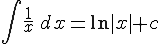So for the example you gave: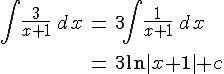Hope that doesn't look too scary### AppleCore

Posted 26 June 2005 - 10:08 PM

That does look crazzzy to me.. then again, i didnt do ADV maths lol!

### dfx

Posted 26 June 2005 - 11:20 PM

Is that Mod x+1 or big brackets x+1 ?

### Discogirl17

Posted 27 June 2005 - 01:14 PM

Its mod (x+1) not mod (x) +1
Is that what you were asking?

### George

Posted 27 June 2005 - 02:14 PM

Yes, it's the absolute value of (x+1), since ln isn't defined for negative numbers.

### dfx

Posted 27 June 2005 - 11:54 PM

erm... and how would you go about solving it?I don't understand why its an absolute value, is it cause the asymptote (presumably) never crosses the ordinate into the negative zone?

### Steve

Posted 28 June 2005 - 10:27 AM

Just in case, the absolute value of a number just means that if it is negative, you make it positive, eg |-2| = 2

The reason its ln|x+1| is because the function ln only has positive real numbers in its domain. Think about the graph of y=ln(x), ie ln(x) is undefined for x0.

As an example, consider the equation: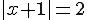Now for the equation to hold, x+1 can be -2 or 2. So x = -3 or x = 1 is the solution.

If you want to find the area under the curve of y = 1/x however, you just have to sub in the limits as usual into ln|x|. For example, the area between x = 1 and x = 3: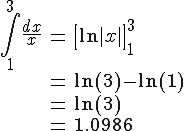So the area is 1.0986 square units

### The Wedge Effect

Posted 28 June 2005 - 04:15 PM

QUOTE(Steve @ Jun 28 2005, 11:27 AM)
Just in case, the absolute value of a number just means that if it is negative, you make it positive, eg |-2| = 2

The reason its ln|x+1| is because the function ln only has positive real numbers in its domain. Think about the graph of y=ln(x), ie ln(x) is undefined for x0.

As an example, consider the equation:Now for the equation to hold, x+1 can be -2 or 2. So x = -3 or x = 1 is the solution.

If you want to find the area under the curve of y = 1/x however, you just have to sub in the limits as usual into ln|x|. For example, the area between x = 1 and x = 3:So the area is 1.0986 square units*Stares blankly, drool dripping, as if I am braindead*I'll probs be doing that stuff in uni anyway.

### dfx

Posted 28 June 2005 - 11:04 PM

Hmmm.. oky doke.. thanks muchos.

Em, what about areas under y = a^x, say 2^x ? I hate learning this trapezium rule so I'm lookin for other methods lol. Thanks.### George

Posted 29 June 2005 - 08:29 AM

Apparantly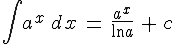I had to look that up in my calculus textbook though; it's not something I've really met yet.

### dfx

Posted 29 June 2005 - 01:52 PM

You're amazing. ThanksNow for some show off to the teacher